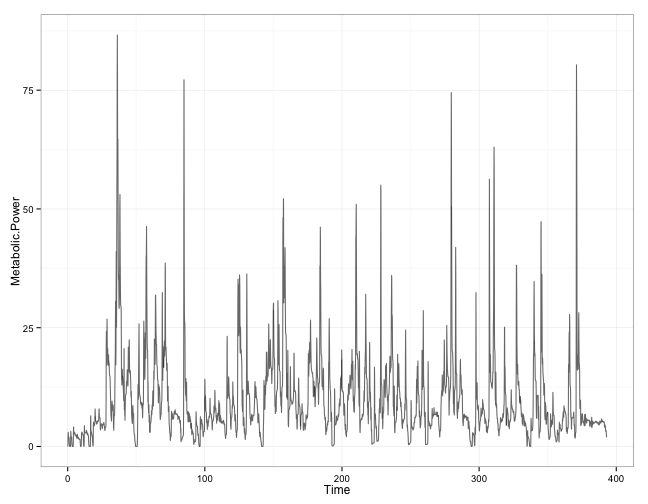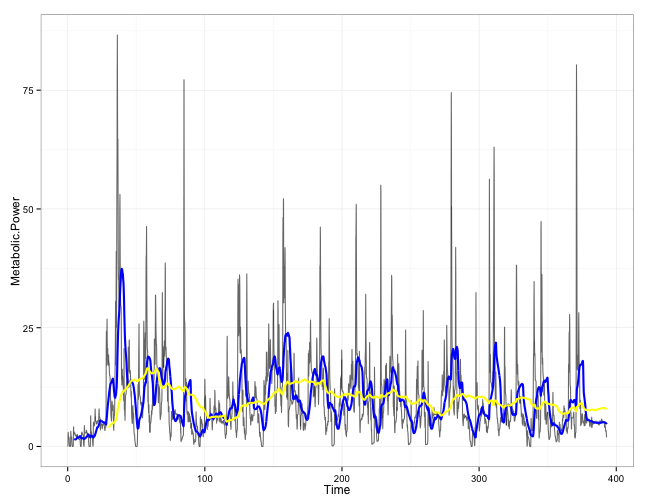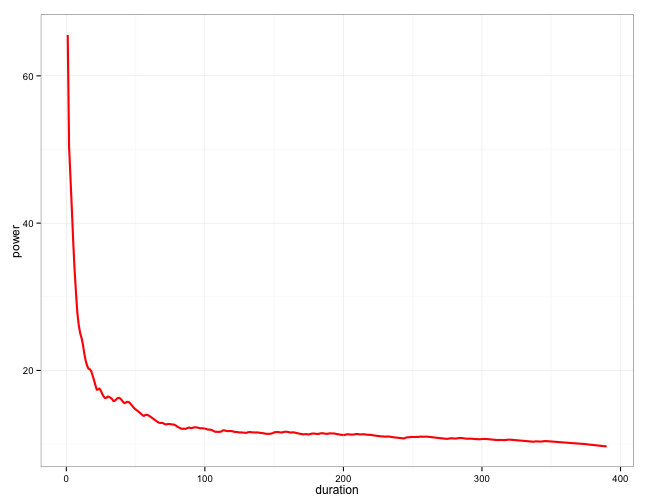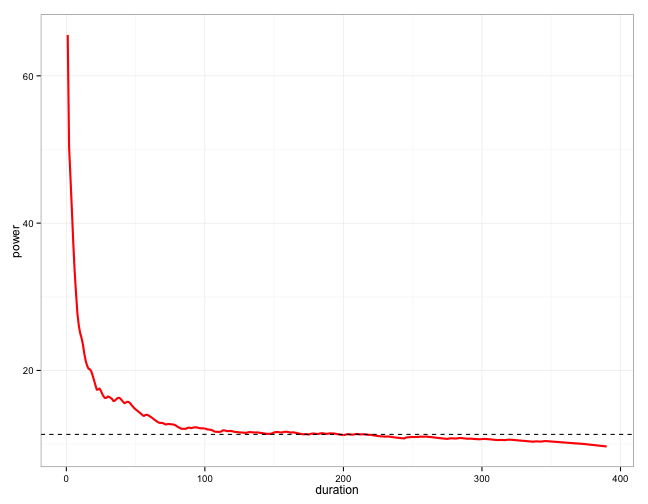## Thursday, March 6, 2014

### Analysis of Metabolic Power data using Power-Duration profile in team sports

Analysis of Metabolic Power data using Power-Duration profile in team sports

# Analysis of Metabolic Power data using Power-Duration profile in team sports

## Introduction

This is the idea I got from the Training and Racing with a Powermeter book by Hunter Allen and Andrew Coggan. It is an excellent and must read book on cycling, but also great book about endurance training in general.

The idea behind Power-Duration profile is that we collect instantenous Metabolic Power (in this case using GPS and tracking players) over certain period of time (e.g. one to two months duration each) and try to find maximal average MP of different durations (e.g. 5sec, 30sec, 60sec, 5min, 20min, 40min). Then we plot this and we get some insight on power cababilities of a given individual over a given time.

If we repeat this process for later period, we can compare change of the profile or selecte spots (5sec, 30sec, 5min and so forth). Although this is expression of one's capacities, by the rule of big numbers and semi-stable weekly training structures in team sports, we can gain some insights on change and individual capacities WITHOUT performing any formal tests.

## Example with Catapult data

I will use the same data set as I have used in Real-Time Fatigue Monitoring using Metabolic Power and CP/W' (I suggest re-reading that one first to refresh the concepts such as Critical Power and Critical Velocity). You can download the data set HERE

The duration of the data sample is short (~400sec, with 10Hz sampling). The analysis should involve lot longer data-sets (couple of weeks of data), but making it fast in R would involve some C++ coding, or using faster algorythm than I have used here.

Here is the data and how it looks like

``````library(ggplot2)

sampling <- 1/10

# Load CVS data from Catapult Export File, Sampling = 10Hz
stringsAsFactors = FALSE)

# Clear up the data
Catapult.data\$X <- NULL

# Create time vector, since we know sampling frequency of 10Hz
Catapult.data\$Time <- seq(from = 0, by = sampling, length.out = length(Catapult.data\$Metabolic.Power))

# Visualize the data using ggplot2
gg <- ggplot(Catapult.data, aes(x = Time, y = Metabolic.Power))
gg <- gg + geom_line(color = "black", alpha = 0.6, size = 0.5)
gg <- gg + theme_bw()
gg
``````The fist step in establishing Power-Duration profile is to calculate moving averages (MA) across the data. In the example below I have created moving averages of 5sec and 30sec duration. As you can see the are 'smoothing' the data (this procedure is sometimes used to filter the noise)

``````gg <- ggplot(Catapult.data, aes(x = Time, y = Metabolic.Power))
gg <- gg + geom_line(color = "black", alpha = 0.6, size = 0.5)

gg <- gg + geom_line(y = filter(Catapult.data\$Metabolic.Power, rep(1, 5/sampling),
sides = 1)/(5/sampling), color = "blue", size = 1)

gg <- gg + geom_line(y = filter(Catapult.data\$Metabolic.Power, rep(1, 30/sampling),
sides = 1)/(30/sampling), color = "yellow", size = 1)

gg <- gg + theme_bw()
gg
``````You can see that MA 'smoothens' the data - the longer the movign window (i.e. 30sec to 5sec) the 'smoother' the data.

The next step is to take out the maximum from the smoothed data. For the sake of example I will pull out maximum of the raw MP, maximum of 5sec MA and maximum of 30sec MA.

``````sprintf("Raw signal maximum: %.2f W", max(Catapult.data\$Metabolic.Power, na.rm = TRUE))
``````
``````##  "Raw signal maximum: 86.62 W"
``````
``````
sprintf("5sec MA signal maximum: %.2f W", max(filter(Catapult.data\$Metabolic.Power,
rep(1, 5/sampling), sides = 1)/(5/sampling), na.rm = TRUE))
``````
``````##  "5sec MA signal maximum: 37.40 W"
``````
``````
sprintf("30sec MA signal maximum: %.2f W", max(filter(Catapult.data\$Metabolic.Power,
rep(1, 30/sampling), sides = 1)/(30/sampling), na.rm = TRUE))
``````
``````##  "30sec MA signal maximum: 16.46 W"
``````

These represent maximal MP over 5sec window and 30sec window. We know from physiology that the longer the duration one can produce less power. Hence, the 5sec max power is higher than 30sec max power.

The next step is to calculate maximums for different MAs over a signal. We can create a for loop and pull out maximums for different time windows.

Here is the code and graphical representation

``````# Define the increase in time windows. The lower is slower, but more
# precise. In longer data sets 5-10sec might be needed. Here (since it is a
# small data set) we use 1
step.value <- 1

# Create a variable to store maximums
power.duration.profile <- data.frame(power = 0, duration = seq(from = 1, to = 390,
by = step.value))

for (i in seq_along(power.duration.profile\$duration)) {
seconds <- power.duration.profile\$duration[i]
power.duration.profile\$power[i] <- max(filter(Catapult.data\$Metabolic.Power,
rep(1, seconds/sampling), sides = 1)/(seconds/sampling), na.rm = TRUE)
}

# Draw the curve
gg <- ggplot(power.duration.profile, aes(x = duration, y = power))
gg <- gg + geom_line(color = "red", size = 1)
gg <- gg + theme_bw()
gg
``````As we can see from the graph there is inverse relationship between Power and duration. This is a common output.

Let's write a function to calculate CP/W from this data set (see more in Rationale and resources for teaching the mathematical modeling of athletic training and performance).

``````criticalPower <- function(data) {
output <- list(CP = 0, W = 0)

duration <- data\$duration
power <- data\$power

duration <- 1/duration

model1 <- lm(power ~ duration)

output\$CP <- coef(model1)[]
output\$W <- coef(model1)[]

criticalPower <- output
}
``````

And we apply this function to our curve (Power-Duration profile)

``````print(criticalPower(power.duration.profile))
``````
``````## \$CP
##  11.31
##
## \$W
##  74.24
``````

In this case CP is ~11W. PLEASE note that this CP/W analysis should come from real testing data (testing capacities vs. expressing them as shown here). Anyway, if we create this Power-Duration profile over a longer period, we can assume that during that period the athlete gave his best effort over a given duration.

To wrap this up, I will replot the caluclated Power-Duration curve and add CP line (shown as dashed line).

``````# Calculate the CP
CP <- criticalPower(power.duration.profile)\$CP

# Draw the curve
gg <- ggplot(power.duration.profile, aes(x = duration, y = power))
gg <- gg + geom_line(color = "red", size = 1)
gg <- gg + theme_bw()
gg <- gg + geom_hline(yintercept = CP, linetype = "dashed")
gg
``````If we repeat this for every motnth or two worth of data, we can MAYBE get CP/W without actually testing players with formal test.